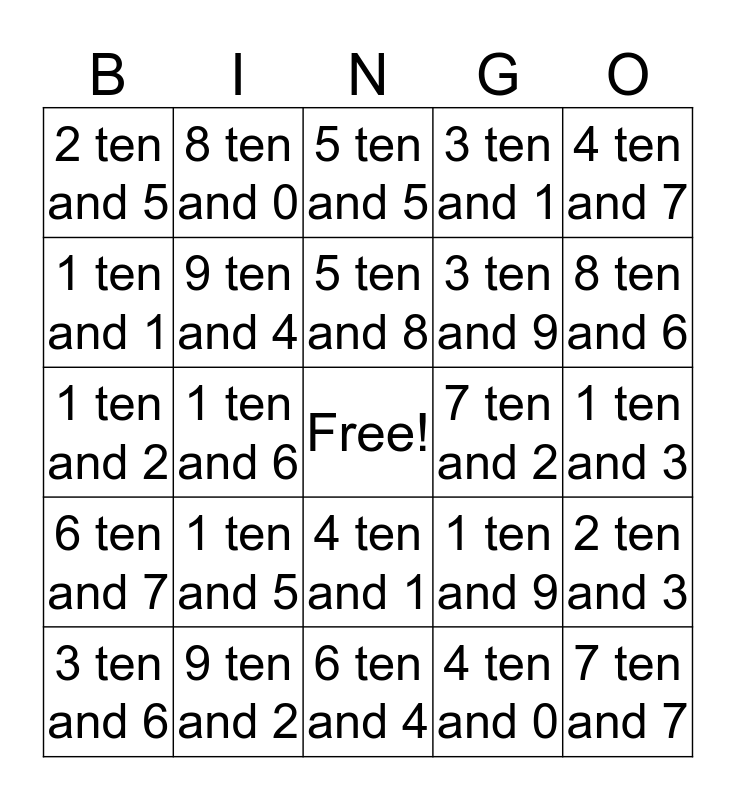# Ten BingoThis bingo card has a free space and 24 words: 1 ten and 5, 9 ten and 2, 5 ten and 8, 3 ten and 6, 4 ten and 7, 2 ten and 5, 1 ten and 1, 8 ten and 0, 7 ten and 2, 4 ten and 1, 3 ten and 9, 1 ten and 2, 1 ten and 9, 2 ten and 3, 9 ten and 4, 4 ten and 0, 1 ten and 3, 5 ten and 5, 8 ten and 6, 1 ten and 6, 7 ten and 7, 6 ten and 7, 3 ten and 1 and 6 ten and 4.

## Play Online